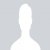How to calculate the defect density in software productsBY anju.r
5 years ago
Drupal-Technical

Defect density could be defined as the value of the total defects which are known to the size of the software product calculated. i.e. Defect Density= Total defects/Size

For better understanding, consider the following example.

Suppose you have a software product which has been integrated with the 4 modules and you found the following bugs in each of the modules.

• Module 1 = 20 bugs
• Module 2 = 30 bugs
• Module 3 = 50 bugs
• Module 4 = 60 bugs
• And the total line of code for each module is

• Module 1 = 1200 LOC
• Module 2 = 3023 LOC
• Module 3 = 5034 LOC
• Module 4 = 6032 LOC

Then, we calculate defect density as

Total bugs = 20+30+50+60 = 160

Size= 15289 LOC

``````
Defect Density =  160/15289 = 0.01046504 defects/loc = 10.465 defects/Kloc
``````

We can use defect density to calculate the following:

1. We can predict the remaining defect in the software product by using the defect density.
2. We can determine whether our testing is sufficient before the release.
3. We can ensure a database of standard defect densities.

Hope this gives you a good understanding of Defect Density.

## RELATED ARTICLEmore_horiz
close

on 01st January 2008 / by webmastermore_horiz
close

on 16th January 2008 / by webmaster